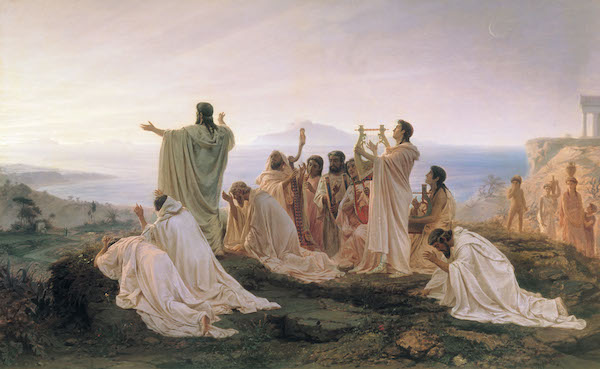# Primitive PythagoreansThe Pythagoreans were students of Pythagoras' school of thought.They subscribed to Pythagoreanism,a philosophy that was dominated by mathematics and mysticism.

A Pythagorean triple consists of three positive integers $(a,b,c)$ such that $a^2+b^2=c^2$.

A triple is said to be primitive if $a$,$b$ and $c$ are co-prime to each other( $GCD(a,b,c)=1$ ).

There are $16$ primitive triples with $c \leq 100$ and $a as shown below.

$( 3, 4, 5 ),( 5, 12, 13),( 8, 15, 17),( 7, 24, 25)$

$(20, 21, 29),(12, 35, 37),( 9, 40, 41),(28, 45, 53)$

$(11, 60, 61),(16, 63, 65),(33, 56, 65),(48, 55, 73)$

$(13, 84, 85),(36, 77, 85),(39, 80, 89),(65, 72, 97)$

Find the number of primitive Pythagorean triples with $c \leq 10^6$ and $a < b < c$ ?

Details and assumptions

• You may assume that the triplets $(a,b,c)$ and $(b,a,c)$ are the same when counting.
• This was inspired by a projecteuler problem
• For more about Pythagoreans and irrational numbers take a look at Peter's note on the discovery of irrational numbers.
• There is a great interactive tutorial on pythagorean triples here.
×

Problem Loading...

Note Loading...

Set Loading...